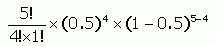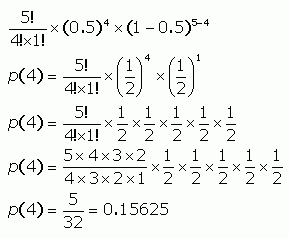SEARCH HOMEMath Central Quandaries & QueriesQuestion from Natasha, a student: well it is p(4) =  5! ------      X (0.5) ^4 X (1- 0.5)^5-4 = 0.15625 4!(5-4)! Now the ^ is to the power of (I believe) Its written in the book as (1-0.5)4 (but the 4 is little and on the top right corner of the first bracket now the second ^5-4 is also little on the top right corner of the 2nd bracket. This is an equation from my text book but for the life of me I cannot figure out how to solve it.  If you can give me step by step procedure I'd like that.  The answer is also there I just don't know how to get to the answer.  Thank you.Hi Natasha. Let me rewrite that using some equation software:Now presuming I have read your question correctly, here are the steps I would use:Begin by simplifying what's in the parentheses. Note that there are implied parentheses on that exponent expression 5-4, so simplify that too. I prefer to use fractions rather than decimals, so I'll convert to fraction form. Next, expand (or simplify) the exponents. (½)4 is four factors of what is in the parentheses. Remember that 5! (read as "five factorial") is just 5 times 4 times 3 all the way down to 2. So expand the factorials so you can see the multiplication they are made from. Then you can cancel out common factors on the tops and bottoms and simplify them. This gives the final fraction of 5/32, which is equal to 0.15625.

Hope this helps,
Stephen La Rocque.Math Central is supported by the University of Regina and The Pacific Institute for the Mathematical Sciences.